Pythagorean Theorem Math Video for Kids | 6th, 7th & 8th Grade
1%
It was processed successfully!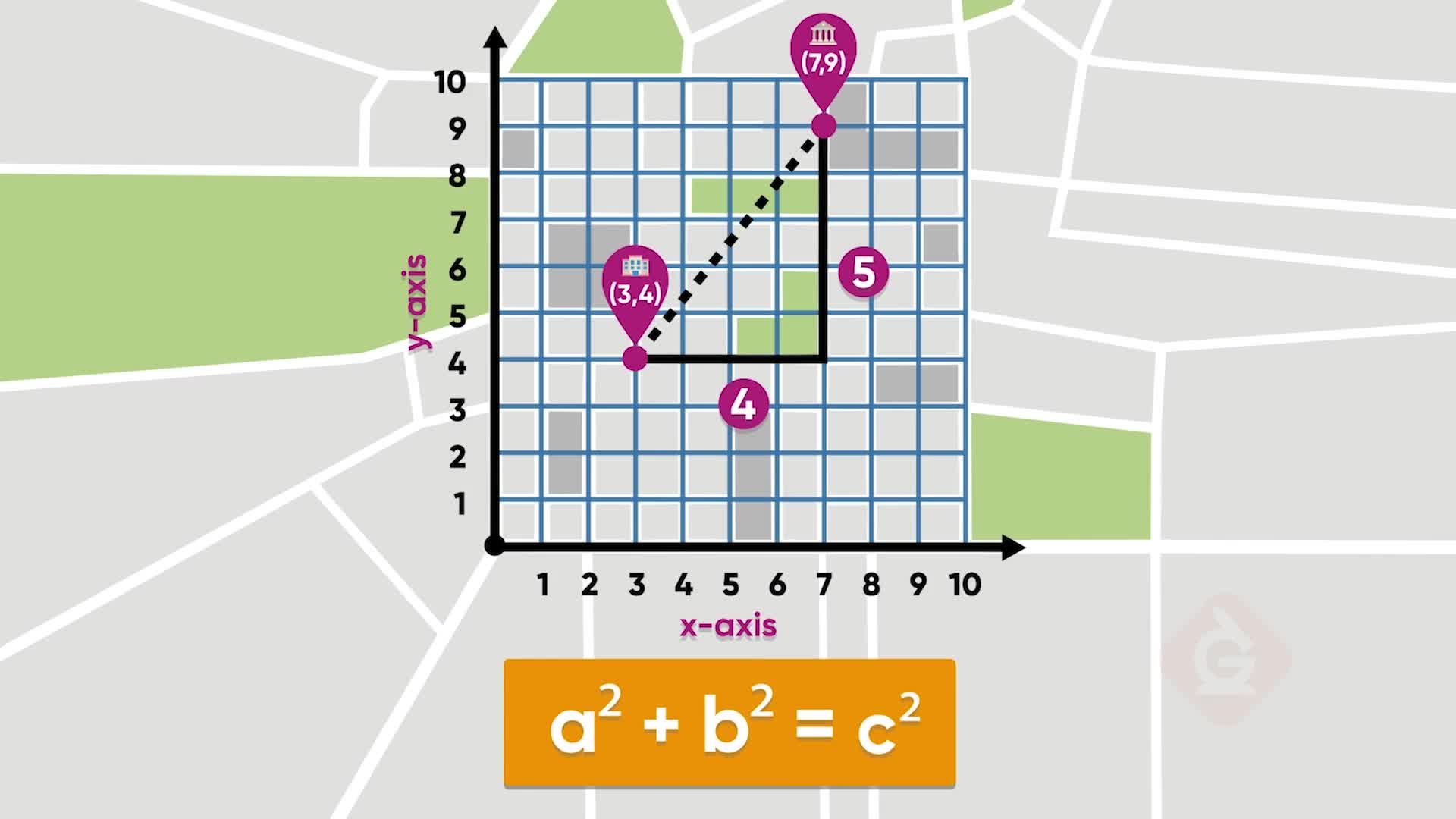WHAT IS THE PYTHAGOREAN THEOREM?

The Pythagorean Theorem is a formula that relates the lengths of the legs and the length of the hypotenuse in a right triangle. The formula is a2+b2=c2. You can use the formula to find a missing side length. You can also use the formula to see if a given set of side lengths could form a right triangle.

To better understand the Pythagorean Theorem…

WHAT IS THE PYTHAGOREAN THEOREM?. The Pythagorean Theorem is a formula that relates the lengths of the legs and the length of the hypotenuse in a right triangle. The formula is a2+b2=c2. You can use the formula to find a missing side length. You can also use the formula to see if a given set of side lengths could form a right triangle. To better understand the Pythagorean Theorem…

## LET’S BREAK IT DOWN!

### Birthday Party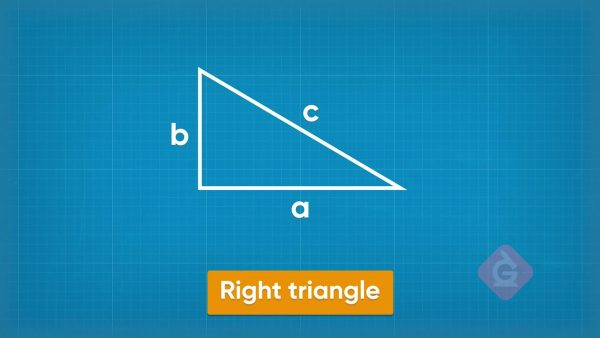The Pythagorean Theorem is a formula that shows how the side lengths of a right triangle are related. The shorter two sides of the triangle are called legs. One leg has length a and the other leg has length b. The longest side is called the hypotenuse. It has length c. The formula is a2+b2=c2. Find the length of c if a = 3 and b = 4. 32 + 42 = 9 + 16 = 25. So, c2 = 25. That means c = 5. Try this one yourself: For a right triangle, find the length of the hypotenuse if a=5 and b=12.

Birthday Party The Pythagorean Theorem is a formula that shows how the side lengths of a right triangle are related. The shorter two sides of the triangle are called legs. One leg has length a and the other leg has length b. The longest side is called the hypotenuse. It has length c. The formula is a2+b2=c2. Find the length of c if a = 3 and b = 4. 32 + 42 = 9 + 16 = 25. So, c2 = 25. That means c = 5. Try this one yourself: For a right triangle, find the length of the hypotenuse if a=5 and b=12.

### Skateboard Ramp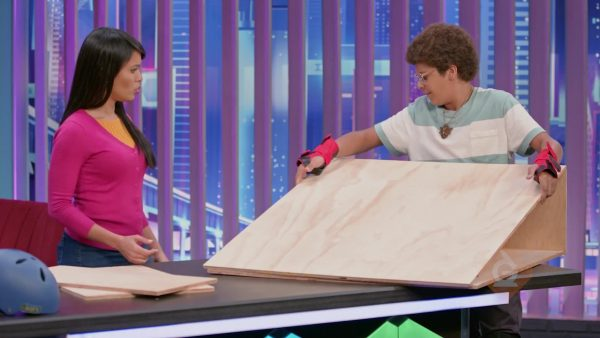The bottom of a skateboard ramp is 100 centimeters long. The height of the ramp is 30 centimeters. What is the length of the ramp part? Substitute 30 and 100 into the formula and find the value of c. 302 + 1002 = 900 + 10,000 = 10,900. Take the square root of 10,900 to find the value of c. The result is irrational, so round to give an approximate answer. c ≈ 104.4 centimeters. The ramp part of the skateboard ramp is approximately 104.4 centimeters long. Try this one yourself: For a right triangle, find the length of the hypotenuse if the base is 6 m and the height is 7 m. Use a calculator, and round your answer to the nearest tenth.

Skateboard Ramp The bottom of a skateboard ramp is 100 centimeters long. The height of the ramp is 30 centimeters. What is the length of the ramp part? Substitute 30 and 100 into the formula and find the value of c. 302 + 1002 = 900 + 10,000 = 10,900. Take the square root of 10,900 to find the value of c. The result is irrational, so round to give an approximate answer. c ≈ 104.4 centimeters. The ramp part of the skateboard ramp is approximately 104.4 centimeters long. Try this one yourself: For a right triangle, find the length of the hypotenuse if the base is 6 m and the height is 7 m. Use a calculator, and round your answer to the nearest tenth.

### Dog Ramp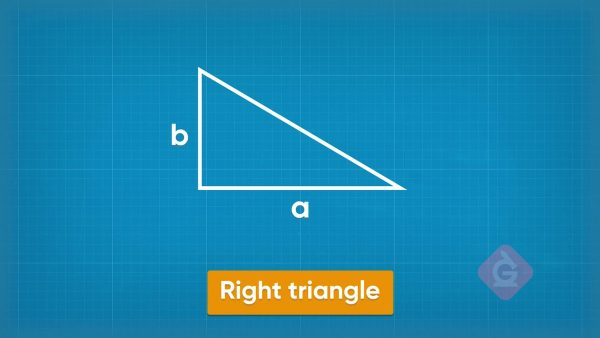The design for a dog ramp says that the longest side is 5 feet long and the base is 4 feet long. What is the height of the ramp? Substitute those two lengths into the formula. You can substitute 4 for either a or b. a2+42=52, so a2+16=25. Subtract 16 from both sides to isolate the a2. a2=9. Since 3×3=9, a=3. The height of the dog ramp is 3 feet. Try this one yourself: One leg of a right triangle is 6 feet long. The hypotenuse is 10 feet long. What is the length of the other side?

Dog Ramp The design for a dog ramp says that the longest side is 5 feet long and the base is 4 feet long. What is the height of the ramp? Substitute those two lengths into the formula. You can substitute 4 for either a or b. a2+42=52, so a2+16=25. Subtract 16 from both sides to isolate the a2. a2=9. Since 3×3=9, a=3. The height of the dog ramp is 3 feet. Try this one yourself: One leg of a right triangle is 6 feet long. The hypotenuse is 10 feet long. What is the length of the other side?

### Beehouse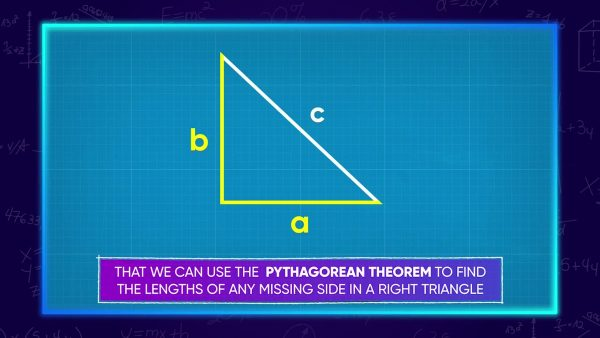If you have a right triangle, then the side lengths make this equation true: a2+b2=c2. Another mathematical fact is that, if a2+b2=c2, then the triangle is a right triangle. For example, three pieces of wood are cut to lengths of 8 centimeters, 15 centimeters, and 17 centimeters. Can these three lengths fit together to make a bee house in the shape of a right triangle? The longest side is 17, so set c=17. Set a=8 and b=15 for the legs. Squaring these numbers, we get a2=64, b2=225, and c2=289. Then, the sum 64+225 is 289. Since a2+b2 has the same value as c2, that means the three pieces of wood can form a right triangle. Try this one yourself: Can the lengths 7 centimeters, 24 centimeters, and 25 centimeters form a right triangle?

Beehouse If you have a right triangle, then the side lengths make this equation true: a2+b2=c2. Another mathematical fact is that, if a2+b2=c2, then the triangle is a right triangle. For example, three pieces of wood are cut to lengths of 8 centimeters, 15 centimeters, and 17 centimeters. Can these three lengths fit together to make a bee house in the shape of a right triangle? The longest side is 17, so set c=17. Set a=8 and b=15 for the legs. Squaring these numbers, we get a2=64, b2=225, and c2=289. Then, the sum 64+225 is 289. Since a2+b2 has the same value as c2, that means the three pieces of wood can form a right triangle. Try this one yourself: Can the lengths 7 centimeters, 24 centimeters, and 25 centimeters form a right triangle?

## PYTHAGOREAN THEOREM VOCABULARY

Legs
The shorter two sides of a right triangle, often denoted by a and b.
Hypotenuse
The longest side of a right triangle, often denoted by c.
Pythagorean Theorem
In a right triangle, the square of the longest side is equal to the sum of the squares of the shorter two sides. This is represented by the formula a2 + b2= c2.
Square root
A value that, when multiplied by itself, gives the number.
Irrational number
A number whose decimal places go on forever with no pattern.
Converse of the Pythagorean Theorem
If three lengths make the formula a2 + b2= c2 true, then the three lengths can form a right triangle.

## PYTHAGOREAN THEOREM DISCUSSION QUESTIONS

### When can you use the Pythagorean Theorem?

When I know two side lengths in a right triangle, and I want to calculate the missing side length.

### How is squaring, as in a2, different from doubling, as in 2a?

a2=a⋅a, whereas 2a=a+a. For example, 32=9 and 3×2=6.

### How would you find the length of the longest side of a triangle if the shorter two sides measure 6 units and 8 units?

Substitute these values into the Pythagorean Theorem to get 62+82=c2. Simplify to 100=c2, and then take the square root to get 10 units.

### How would your calculations change if one leg measures 6 units and the hypotenuse measures 8 units?

I can still substitute 6 for a, but now I substitute 8 for c instead of b. The formula 62+b2=82 yields 36+b2=64, or b2=28. The exact value is square root of 28, which is about 5.3 units, rounded to the nearest tenth.

### How can the Pythagorean Theorem help you find a distance on the coordinate plane?

I draw a triangle with the hypotenuse along the diagonal line that I want to measure. I count the lengths of the legs and substitute them for a and b in the Pythagorean Theorem. Then I can solve for c to find its length.
X

## Success

We’ve sent you an email with instructions how to reset your password.
Ok

## Choose Your Free Trial Period### 7 DaysContinue to Lessons### 90 DaysGet 90 days free by inviting other teachers to try it too.

Share with Teachers

## Get 90 Days Free

### By inviting 4 other teachers to try it too.

4 required

Skip, I will use a 7 days free trial

## Thank You!

Enjoy your free 90 days trial